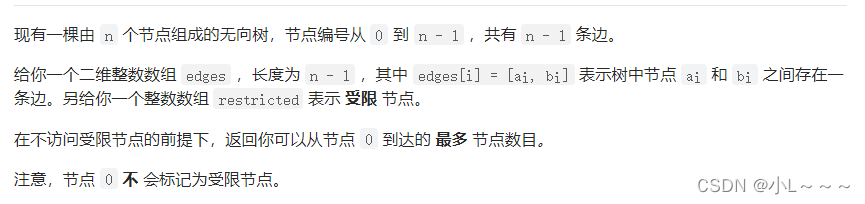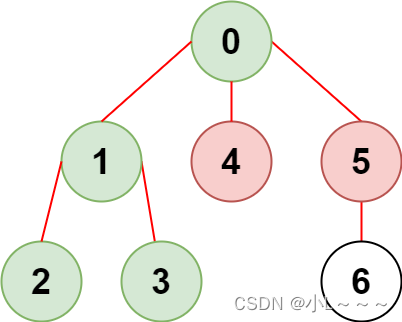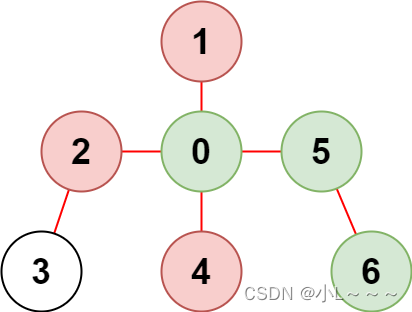## 每日一题-力扣（leetcode）2368. 受限条件下可到达节点的数目

news/2023/6/7 1:07:59

### 题目描述#### 示例 1：#### 示例 2：#### AC代码

``````class Solution {
public:bool visited = {false};int reachableNodes(int n, vector<vector<int>>& edges, vector<int>& restricted) {int ans = 0;queue<int>q;for(auto i : restricted) visited[i] = true;vector<int>G, a;for(int i = 0; i < edges.size(); i++) {a = edges[i];G[a].push_back(a);G[a].push_back(a);}//    for(int i = 0; i < n; i++) {//        cout << "i=" << i << " ";//        for(auto j : G[i]) cout << j << " ";//        cout << endl;//    }q.push(0);while(!q.empty()) {int top = q.front();q.pop();for(auto i : G[top]) {if(visited[i]) continue;q.push(i);ans++;visited[i] = true;}}return ans == 0 ? 1 : ans; // 如果根节点和其他任何结点都有限制则返回1}
};
``````